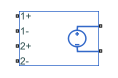# PVCVS2

Polynomial voltage-controlled voltage source with two controlling inputs

• Library:
• Simscape / Electrical / Additional Components / SPICE Sources

•## Description

The PVCVS2 (Two-Input Polynomial Voltage-Controlled Voltage Source) block represents a voltage source whose output voltage value is a polynomial function of the voltages across the pairs of controlling input ports. This equation describes the voltage across the source as a function of time:

`${V}_{out}={p}_{1}+{p}_{2}*{V}_{in1}+{p}_{3}*{V}_{in2}+{p}_{4}*{V}_{in1}^{2}+{p}_{5}{V}_{in1}*{V}_{in2}+{p}_{6}*{V}_{in2}^{2}+\dots$`

where:

• Vin1 is the voltage across the first pair of input ports.

• Vin2 is the voltage across the second pair of input ports.

• p is the Polynomial coefficients parameter value.

## Ports

### Conserving

expand all

Electrical conserving port associated with the PVCVS2 positive input voltage of the first controlling input.

Electrical conserving port associated with the PVCVS2 negative input voltage of the first controlling input.

Electrical conserving port associated with the PVCVS2 positive input voltage of the second controlling input.

Electrical conserving port associated with the PVCVS2 negative input voltage of the second controlling input.

Electrical conserving port associated with the PVCVS2 positive output voltage.

Electrical conserving port associated with the PVCVS2 negative output voltage.

## Parameters

expand all

The polynomial coefficients that relate the input voltage to the output voltage, as described in the preceding section.

## Version History

Introduced in R2009a